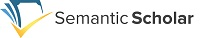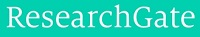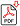Search for Articles:

 Registered AtDifferential Equations Which are Substitutable to Bessel Differential Equation

Abstract: Differential equations in which p is an integer is known as p order of Bessel equation. This differential equation is one of the most important differential equations in applied mathematics. The answers or solutions of this equation are known as Bessel equation. The first solution to this equation is: Which is called Bessel function of the first kind. If p is non-integer, the second solution to the above mentioned equation is: So, the general solution to the above equation is: However, if p is an integer, then the second solution with the first solution is not linearly independent but linearly dependent; thus, the second solution is determined by the following relation which is called Bessel function of the second-order. And with the first solution, it is linearly independent, so the general solution in both cases is: All the differential equation of the second-order that can be substituted into Bessel equation due to some appropriate replacements, we can easily find out their solution by Bessel equation.

Keywords: Bessel equation, Bessel functions of the first and second kind, differential equations of the second-order, differential equations of the second-order solution

Country: Afghanistan, Subject Area: Mathematics

Pages: 448 - 452

Edition: Volume 8 Issue 8, August 2019